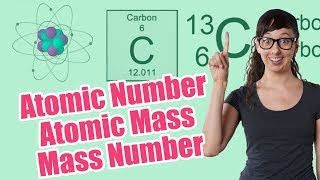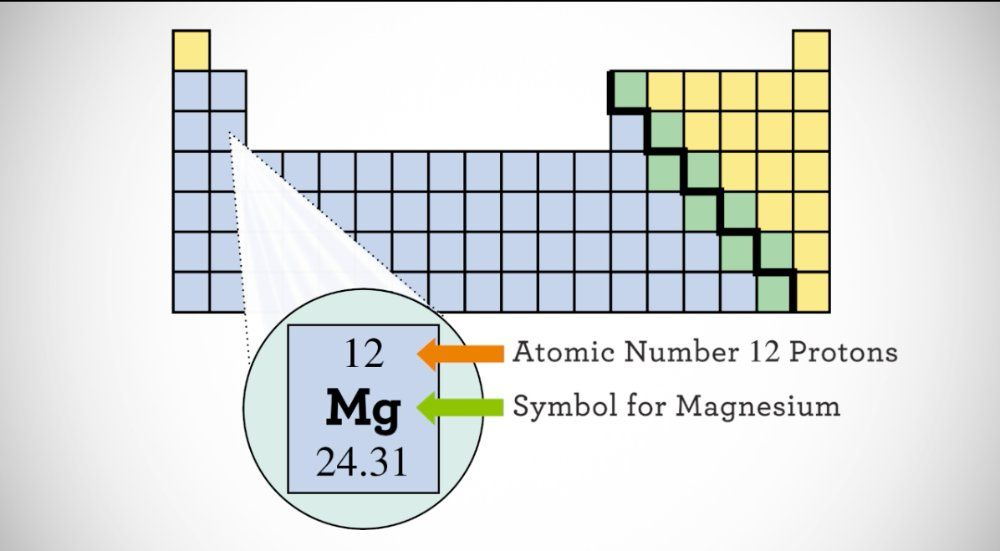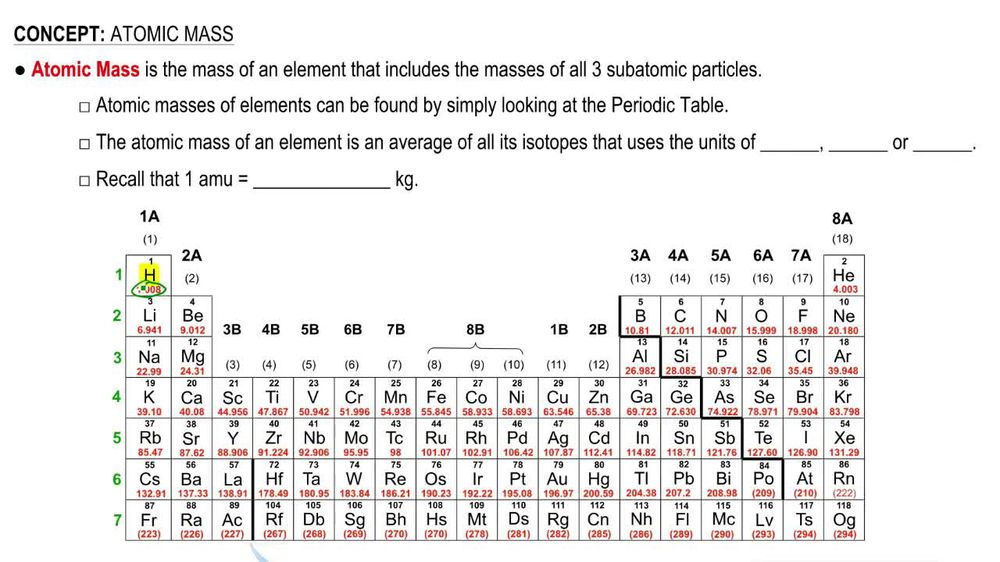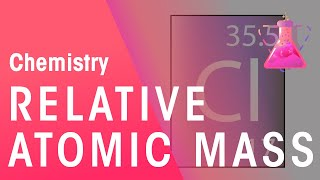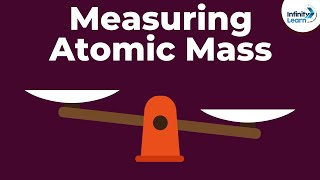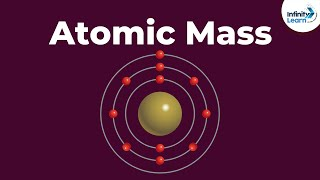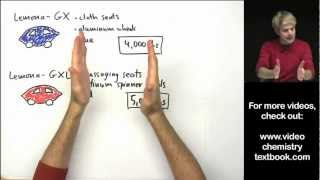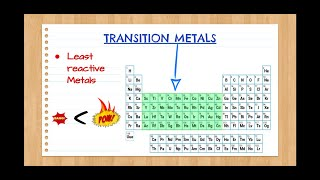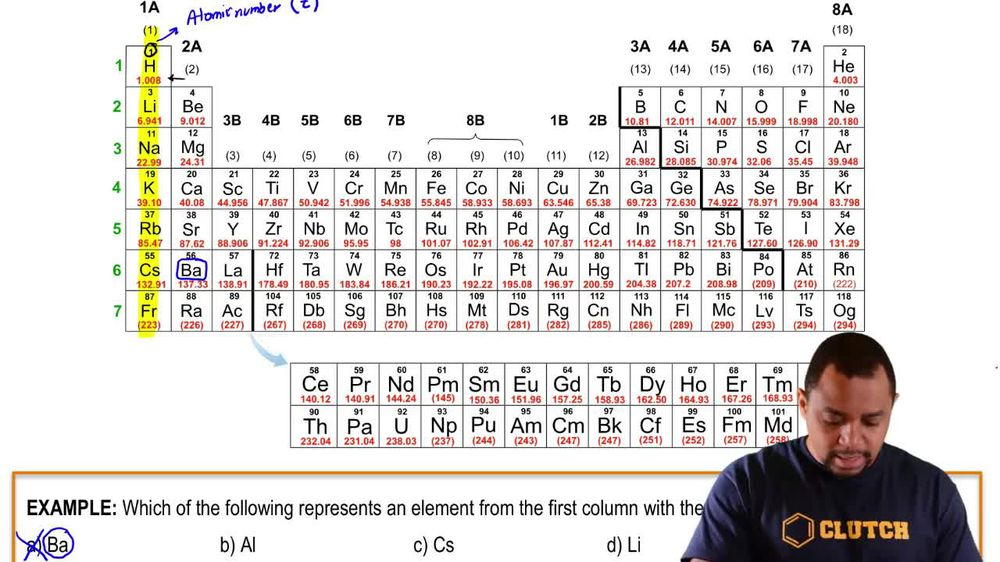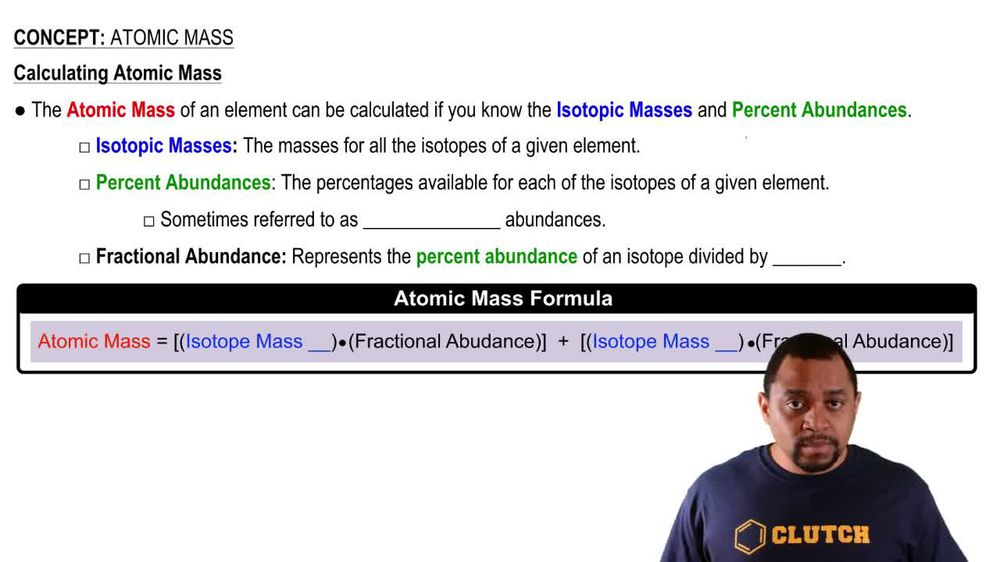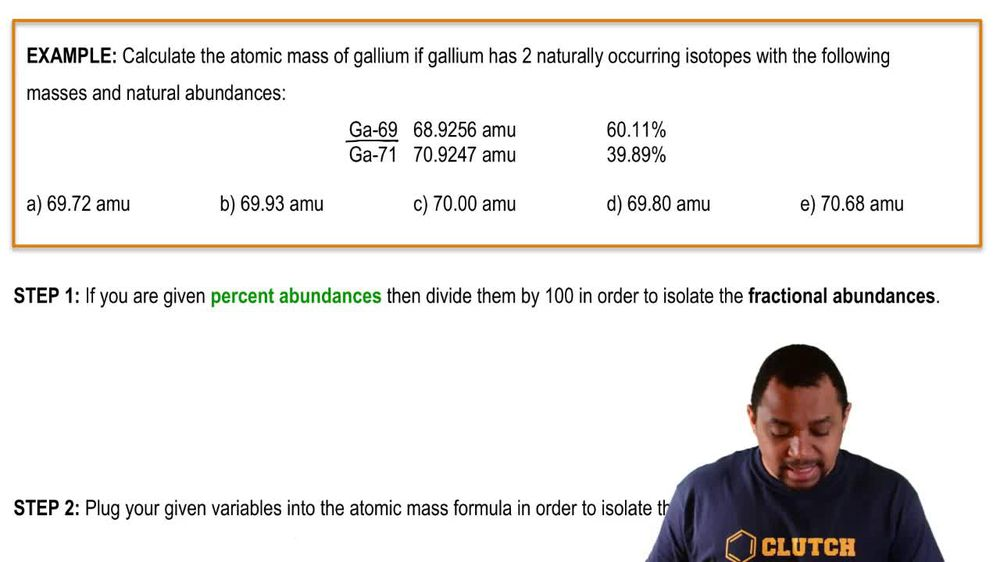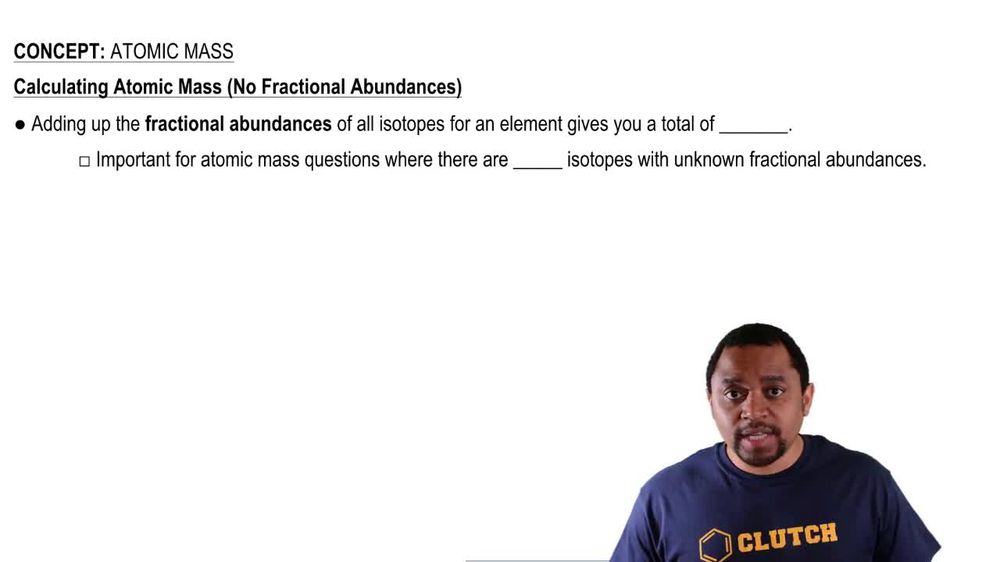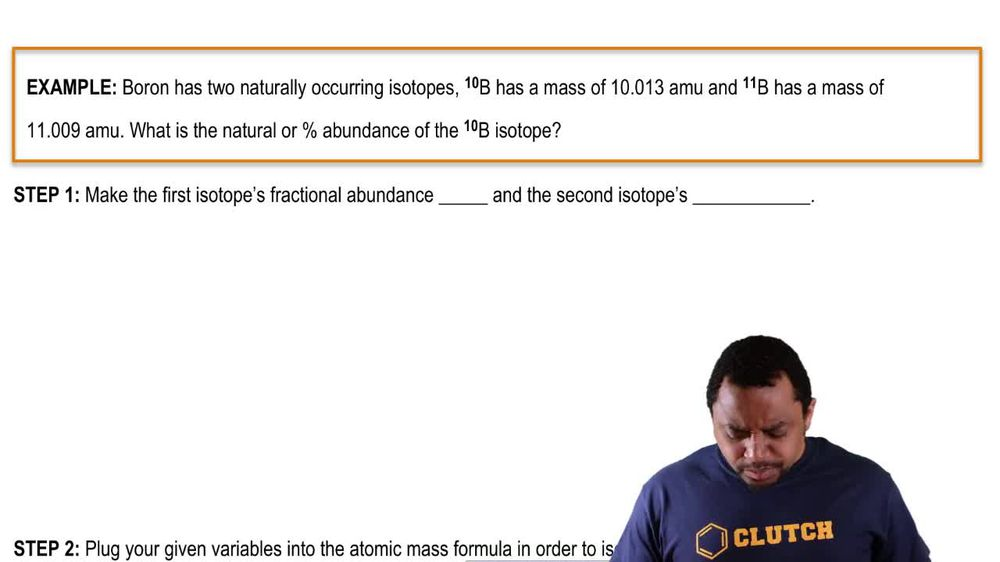Start typing, then use the up and down arrows to select an option from the list.
1. 2. Atoms & Elements2. Atomic Mass
Problem

# Naturally occurring chlorine is composed of two isotopes: 75.76% Cl-35 (mass 34.9688 amu) and 24.24% Cl-37 (mass 36.9659 amu). Naturally occurring oxygen is composed of three isotopes: 99.757% O-16 (mass 15.9949 amu), 0.038% O-17 (mass 16.9991 amu), and 0.205% O-18 (mass 17.9991 amu). The compound dichlorine monoxide is composed of two chlorine atoms and one oxygen atom bonded together to form the Cl2O molecule. How many Cl2O molecules of different masses naturally exist? Give the masses of the three most abundant Cl2O molecules.

Relevant Solution4m
Play a video:
Hello everyone today. We are being given the following problem and asked to solve for it. A zinc wire with 1.27 millimeter radius weighs 17 kg, calculate the overall resistance of the wire if it has a resistance of 1.39 per kilometer. So our first step, the very first thing that we want to do is we want to calculate our volume and we can do that by using the equation. That volume is equal to our mass over our density. And so we have the density of zinc From a reference table being equal to 7.133 g over centimeters cubed. And so we have the mass Which is equal to R kilograms. What we're gonna do is we're gonna take this and we're going to change that mass into regular grams. So we're gonna take our 17 kg. And multiply the conversion factor that 1000 g is equal to one kg When our units canceled out. Were given 1.7 times 10 to the 4th g. We can then plug this back into our original volume equation says that volume is equal to our mass over our density. And so our masses we just calculated is 1.7 times 10 to the 4th g. And our density is that 7.133g per cm cubed Units of g are going to cancel out. And we are going to be left with 2,383. centimeters cubed for our volume. Our second step is going to be to calculate the length of this wire. We can do that by using the equation. That volume is equal to r length times pi R squared. But they're gonna rearrange this equation so that I or the length L or the length can equal volume over pi R squared. Now we're gonna take some units some values that we have in the problem and apply them here. So we have our radius 1.27 mm and we need to convert that into centimeters. And so we're gonna do that over here. We're gonna take our 1.27 millimeters and use the conversion factor that one millimeter is equal to one times 10 to the negative third meters. So that when our units canceled out. We also have to additionally multiply this to get two cm and we can use the additional factor that one m is equal to 100 cm. So that now that our meters are canceled out. We get 0.1-7 cm. We can unplug the sense of the equation of L. On length We have a volume that we calculated or 383.29 cm Cubed. We are then going to divide that by our pi value. Our radius is 0.127 cm. We're gonna square that. That's gonna give us a value of 120.76 centimeters. The 3rd and final step is to calculate our resistance in units of homes. So units of homes and we're doing that, we can take the link that we just got our 120.76 cm and we can use the conversion factor that one m is equal to 100 centimeters and then that one kilometer is equal to m. And then the reference value conversion factor that one km is equal to 1.039 homes. To get a final value of 1.25 times 10 to the negative third homes. And with that we have our final answer. I hope this helped, and until next time.